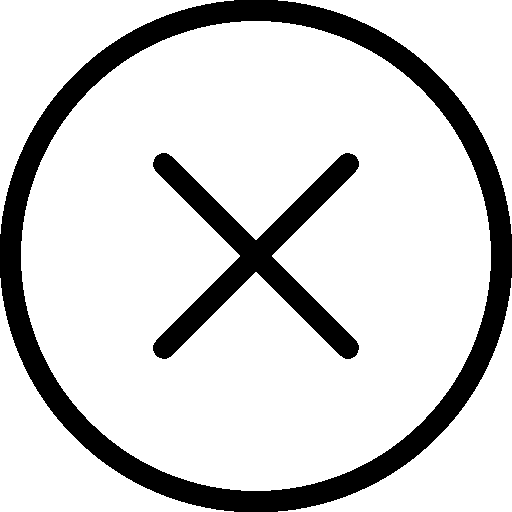• 写文章
• 提问

# python3 re有哪些返回形式？findall(pattern, string, flags=0)

```import re

pattern = re.compile(r'\W+')
result1 = pattern.findall('hello world!')
result2 = pattern.findall('hello world!', 0, 7)

print(result1) #[' ', '!']
print(result2) #[' ']```

finditer(pattern, string, flags=0)

```import re

pattern = re.compile(r'\W+')
result = pattern.finditer('hello world!')
for r in result:
print(r)
# <re.Match object; span=(5, 6), match=' '>
# <re.Match object; span=(11, 12), match='!'>```